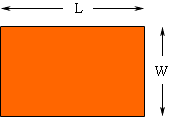Quandaries and Queries I have a room that is rectangular the area is 159.5 feet, the perimeter is 51 feet what would the dimensions of the room. ESTELLA Hi Estella, Draw a diagram and label the lenght L and the width W.The area of a rectangle is the length times the width so LW = 159.5 The perimeter is the distance around the room so L + W + L + W = 51 thus L + W = 25.5 Hence L = 25.5 - W Substitute this value for L into the first equation to get (25.5 - W)W = 195.5 Simplify and solve for W. Penny Go to Math Central